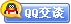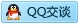##用户名 Email 自动登录 找回密码 密码 会员注册
 VIP会员，3年作业免费下 ！ 奥鹏作业，奥鹏毕业论文检测 新手作业下载教程，充值问题 没有找到答案，请在此处留言！ 2019年12月最新全国统考资料 投诉建议，加盟合作！奥鹏课程积分软件(ver:3.1)

# 北航12春《概率统计》在线作业二发表于 2012-3-14 22:00:44 | 显示全部楼层 |阅读模式谋学网: www.mouxue.com 主要提供奥鹏作业答案，奥鹏在线作业答案，奥鹏离线作业答案以及奥鹏毕业论文，致力打造中国最专业远程教育辅导社区。 一、单选题（共 25 道试题，共 100 分。）V 1.  假设事件A和B满足P(A∣B)＝1，则 A. A、B为对立事件 B. A、B为互不相容事件 C. A是B的子集 D. P(AB)=P(B)       满分：4  分 2.  对于任意两个事件A与B,则有P(A-B)=(). A. P(A)-P(B) B. P(A)-P(B)+P(AB) C. P(A)-P(AB) D. P(A)+P(AB)       满分：4  分 3.  设X与Y是相互独立的两个随机变量，X的分布律为：X=0时，P=0.4；X=1时，P=0.6。Y的分布律为：Y=0时，P=0.4，Y=1时，P=0.6。则必有（ ） A. X=Y B. P{X=Y}=0.52 C. P{X=Y}=1 D. P{X#Y}=0       满分：4  分 4.  一台设备由10个独立工作折元件组成，每一个元件在时间T发生故障的概率为0.05。设不发生故障的元件数为随即变量X,则借助于契比雪夫不等式来估计X和它的数学期望的离差小于2的概率为（　　） A. 0.43 B. 0.64 C. 0.88 D. 0.1       满分：4  分 5.  某单位有200台电话机，每台电话机大约有5%的时间要使用外线电话，若每台电话机是否使用外线是相互独立的，该单位需要安装（ ）条外线，才能以90%以上的概率保证每台电话机需要使用外线时而不被占用。 A. 至少12条 B. 至少13条 C. 至少14条 D. 至少15条       满分：4  分 6.  一批10个元件的产品中含有3个废品，现从中任意抽取2个元件，则这2个元件中的废品数X的数学期望为（　） A. 3/5 B. 4/5 C. 2/5 D. 1/5       满分：4  分 7.  设A、B互不相容，且P(A)>0,P(B)>0则下列选项正确的是（）。 A. P(B/A)>0 B. P(A/B)=P(A) C. P(A/B)=0 D. P(AB)=P(A)*P(B)       满分：4  分 8.  设随机变量的数学期望E（ξ）=μ，均方差为σ，则由切比雪夫不等式，有{P（|ξ-μ|≥3σ）}≤（ ） A. 1/9 B. 1/8 C. 8/9 D. 7/8       满分：4  分 9.  设随机变量X和Y的方差存在且不等于0，则D（X+Y）=D（X）+D（Y）是X和Y（ ） A. 不相关的充分条件，但不是必要条件 B. 独立的充分条件，但不是必要条件 C. 不相关的充分必要条件 D. 独立的充要条件       满分：4  分 10.  设X,Y为两个随机变量，已知cov(X,Y)=0,则必有（）。 A. X与Y相互独立 B. D(XY)=DX*DY C. E(XY)=EX*EY D. 以上都不对       满分：4  分 11.  假设一厂家一条自动生产线上生产的每台仪器以概率0.8可以出厂，以概率0.2需进一步调试，经调试后，以概率0.75可以出厂，以概率0.25定为不合格品而不能出厂。现该厂新生产了十台仪器（假设各台仪器的生产过程相互独立），则十台仪器中能够出厂的仪器期望值为（　） A. 9.5 B. 6 C. 7 D. 8       满分：4  分 12.  把一枚质地均匀的硬币连续抛三次，以X表示在三次中出现正面的次数，Y表示在三次中出现正面的次数与出现反面的次数的差的绝对值，则｛X＝2，Y＝1｝的概率为（　） A. 1/8 B. 3/8 C. 3/9 D. 4/9       满分：4  分 13.  已知P(A)=0.3,P(B)=0.4,P(AB)=0.2,则P（B|A）=________. A. 1/3 B. 2/3 C. 1/2 D. 3/8       满分：4  分 14.  袋内装有5个白球，3个黑球，从中一次任取两个，求取到的两个球颜色不同的概率 A. 15/28 B. 3/28 C. 5/28 D. 8/28       满分：4  分 15.  任何一个随机变量X，如果期望存在，则它与任一个常数C的和的期望为（　） A. EX B. EX＋C C. EX－C D. 以上都不对       满分：4  分 16.  设两个随机变量X与Y相互独立且同分布；P{X=-1}=P{Y=-1}=1/2,P{X=1}=P{Y=1}=1/2,则下列各式中成立的是（）。 A. P{X=Y}=1/2 B. P{X=Y}=1 C. P{X+Y=0}=1/4 D. P{XY=1}=1/4       满分：4  分 17.  电灯泡使用时数在1000小时以上的概率为0.2，求三个灯泡在1000小时以后最多有一个坏了的概率（ ） A. 0.7 B. 0.896 C. 0.104 D. 0.3       满分：4  分 18.  三人独立破译一密码，他们能单独译出的概率分别为1/5,1/3,1/4,则此密码被译出的概率是 A. 2/5 B. 3/4 C. 1/5 D. 3/5       满分：4  分 19.  设随机变量X和Y相互独立，X的概率分布为X=0时，P=1/3；X=1时，P=2/3。Y的概率分布为Y=0时，P=1/3；Y=1时，P=2/3。则下列式子正确的是（ ） A. X=Y B. P{X=Y}=1 C. P{X=Y}=5/9 D. P{X=Y}=0       满分：4  分 20.  利用样本观察值对总体未知参数的估计称为( ) A. 点估计 B. 区间估计 C. 参数估计 D. 极大似然估计       满分：4  分 21.  某车队里有1000辆车参加保险，在一年里这些车发生事故的概率是0.3%，则这些车在一年里恰好有10辆发生事故的概率是（　） A. 0.0008 B. 0.001 C. 0.14 D. 0.541       满分：4  分 22.  如果随机变量X服从标准正态分布，则Y＝－X服从（　) A. 标准正态分布 B. 一般正态分布 C. 二项分布 D. 泊淞分布       满分：4  分 23.  设随机事件A，B及其和事件A∪B的概率分别是0.4，0.3和0.6，则B的对立事件与A的积的概率是 A. 0.2 B. 0.5 C. 0.6 D. 0.3       满分：4  分 24.  设随机变量X和Y独立同分布，记U=X-Y，V=X+Y，则随机变量U与V必然（ ） A. 不独立 B. 独立 C. 相关系数不为零 D. 相关系数为零       满分：4  分 25.  设A,B,C是两两独立且不能同时发生的随机事件，且P(A)=P(B)=P(C)=x,则x的最大值为（）。 A. 1/2 B. 1 C. 1/3 D. 1/4       满分：4  分 谋学网: www.mouxue.com 主要提供奥鹏作业答案，奥鹏在线作业答案，奥鹏离线作业答案以及奥鹏毕业论文，致力打造中国最专业远程教育辅导社区。
 您需要登录后才可以回帖 登录 | 会员注册 本版积分规则 回帖并转播 回帖后跳转到最后一页客服一客服二客服三客服四微信客服扫一扫